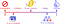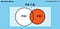# Conditional Probability: Definition, Properties and Examples

Let us learn the theory of conditional probability in context with definition, examples and properties through this blog.

Being a classical concept in probability theory, the conditional probability is one of the prominent approaches of measuring the probability of occurrence of an event, provided that another event has occurred.

# Learning Objectives

*Introduction

*What is the conditional probability?

• Conditional probability of independent events
• Conditional probability of mutually exclusive events
• Chain rule or multiplication rule
• The law of total probability

*Properties of conditional probability

*Examples of conditional probability, and

*Ending notes

# Introduction

First, let’s catch the quick introduction to the concept of probability.

• Can we measure the chances that something will happen?
• How likely that an event will occur?

When we say that there are “20% chances”, we are quantifying some events and use words like impossible, unlikely, even like, likely, and certain to measure the probability.

Probability is simply the measure of the likelihood that an event will occur. And, in the form of a number, the probability is from 0 (impossible) to 1 (certain). The sum of all probabilities of all the events in a sample space is equal to the 1. For example, the probability of event A is the sum of the probabilities of all the sample points in event A and denoted by P(A).Probability’s journey from 0 to 1, Source

Now, consider the example to know the essence of conditional probability, a fair die is rolled, the probability that it shows “4” is 1/6, it is an unconditional probability, but the probability that it shows “4” with the condition that it comes with even number, is 1/3, this is a conditional probability.

# What Is Conditional Probability?

Typically, the conditional probability of the event is the probability that the event will occur, provided the information that an event A has already occurred. This probability can be written as P(B|A), notation signifies the probability of B given A.

In other words, the conditional probability is the probability that an event has occurred, taking into account some additional information about the outcomes of an experiment.

(Must read: Introduction to Probability Distributions)

Mathematically, if the events A and B are not independent events, then the probability of the interaction of A and B (the probability of occurrence of both events) is then given by:

P(A and B) = P(A) P(B|A),

Or it can be written as;

P(A⋂ B)= P(A)P(B|A),

And, from this definition, the conditional probability P(B|A) can be defined as:

P(B|A)= P(A and B)|P(A)Venn diagram for Conditional Probability, P(B|A)

Or, simply;

P(B|A)= P(A⋂ B)P(A), as long as P(A)> 0

(Recommended blog: Importance of Probability in Data Science)

# Conditional Probability of Independent Events

Also, in some cases events, A and B are independent events,i.e., event A has no effect over the probability of event B, that time, the conditional probability of event B given event A, P(B|A), is the essentially the probability of event B, P(B). The formula is given by P(B|A)= P(B)

Or, the conditional probability of two independent events are;

• When given the event A, probability of event B occurring is given by

P(B|A)= P(B)

• And, the given event B, probability of event A occurring is given by

P(A|B)= P(A)

# Conditional Probability of Mutually Exclusive Events

Under the probability theory, the mutually exclusive events are the events that cannot occur simultaneously. In simple words, if one event has already occurred, another event cannot occur at the same time. Therefore, the probability of mutually exclusive events is always zero.

Therefore, P(B|A)= 0 and P(A|B)= 0

# Chain Rule or Multiplication Rule

Let E be an event happening given F be another event that has occurred. In that condition, The formula of conditional probability can be rewritten as :

P(E ⋂ F) = P(E|F) P(F)

This is known as a chain rule or the multiplication rule. Typically, it states that the probability of observing events, E and F, is the product of the probability of observing F event and the probability of observing E given that event F has been observed.

The generalized form of multiplication rule is;

P( E1 ⋂ E2 ⋂….. ⋂En)=P( E1) P(E2 | E1)………P(En | E1…………En-1)

# The Law of Total Probability

The law of total probability is simply the use of the multiplication rule to measure the probabilities in more interesting cases. Suppose the sample space S is segmented into three disjoint events X, Y, Z, then for any event:

P(A)=P(A ⋂ X) +P(A ⋂ Y) +P(A ⋂ Z)

The above equation states that event A is split into three parts, the P(A) is the sum of the probabilities of each part individually. Now using the multiplication rule, the probability of event A can be restated as;

or, P(A)= P(A|X) P(X) +P(A|Y) P(Y) +P(A| Z) P(Z)

This is called the law of total probability.

(Also read: 7 Major Branches of Discrete Mathematics)

# Properties of Conditional Probability

Following are some fundamental properties of conditional properties;

Property 1

Suppose, X and Y be the two events of a sample space S of an experiment, then it can be said that

P(S|Y) = P(Y|Y) = 1

Property 2

Let X and Y are two events of a sample space S, and F is the event such that P(F) ≠ 0, then A and B are any two events of a sample space S and F is an event of S such that P(F) ≠ 0, then;

P((X ∪ Y)|F) = P(X|F) + P(Y|F) — P((X ∩ Y)|F)

Property 3

• In conditional probability, the order of the sets or events matters so;

P(A|B) P(B|A)

• The complement formula holds only in the context of the first argument, there is not any corresponding formula for P(A|B’). Hence,

P(A|B’)1-P(A|B)

But, P(A’|B)=1-P(A|B)

Property 4

The independence of three events or more events: Assuming A, B, C as mutually independent if the product formula holds for

(i) the intersection of all three events, i.e.,

P(A ⋂ B ⋂ C) = P(A) P(B) P(C), and

(ii) for any combination of two of these three events, i.e.,

P(A ⋂ B) = P(A) P(B), and similarly for P(A ⋂ C), P(B ⋂ C).

# Examples of Conditional Probability

In this section, let’s understand the concept of conditional probability with some easy examples;

Example 1

A fair die is rolled, Let A be the event that shows an outcome is an odd number, so A={1, 3, 5}. Also, suppose B the event that shows the outcome is less than or equal to 3, so B= {1, 2, 3}. Then what is the probability of A, P(A), and what is the probability A given B, P(A|B).

So the solution is for P(A),

total sample space= 6,

Total odd number when rolling dice once= 3

Hence, P(A)= Event A/sample space (S)

= |(1, 3, 5)|/S

= 3/6

= 1/2.

And now, the solution for P(A|B), for calculating conditional probability of A given that B has happened. B has the outcomes {1,2,3} and A has {1, 3, 5}. Here (A⋂B)= {1, 3} that are two numbers.

So, P(A|B)= P(A⋂ B)/ B

= 2 / 3.

(Read also: A Fuzzy-Logic Approach In Decision-Making)

Example 2

A coin is tossed three times, sample space, S= {HHH, HHT, HTH, HTT, THH, THT, TTH, TTT}, i.e. 8 elements.

What is the probability of three heads?

Since from the sample space we can say that occurring 3 times head is once only, that is 1 element.

P( getting 3 heads)= 1/8.

If given that an event that shows the first toss was heads, then what is the probability of three heads.

Now, from sample space, let B is the event that shows the first toss is heads;

B= {HHH, HHT, HTH, HTT}, i.e, 4 elements,

A be the event of an occurrence of three heads

A={HHH}

Then (A⋂B)= {HHH}, i.e, 1 element.

Then the P( getting 3 heads given that first toss is heads), or

P(A|B) = P(A⋂B)/B

= 1/4.

Example 3

A die is rolled twice and two numbers are obtained, let X be the outcome of first role and Y be the outcome of the second roll. Given that X+Y=5, what is the probability of X=4 or Y=4?

Assume, A be the event the getting 4 as X or Y, and B be the event of X+Y=7, therefore

A={(4,1), (4,2), (4, 3), (4,4), (4,5), (4,6), (1,4), (2,4), (3,4), (4,4), (5,4), (6,4)}

B={ (1,4),(4,1), (2, 3), (3,2)}

We are interested in finding the probability of A given B

A⋂ B= {(1,4), (4, 1)}

As die is rolled out two times, total sample space= 36

P(A⋂ B)= 2/ 36

P(B)= 4/ 36

So, P(A|B)= P(A⋂ b)/ P(B)

= 2/4, or

= 1/2.

For more examples, check the video that shows how to calculate the conditional probability,

# Ending Notes

What if an individual wants to check the chances of an event happening given that he/she already has observed some other event, F. This is a conditional probability.

(Recommended blog: What is Confusion Matrix?)

However, conditional probability doesn’t describe the casual relationship among two events, as well as it also does not state that both events take place simultaneously. It is the most critical perception in machine learning and probability theory as it enables us to revise our assumptions in the form of new pieces of evidence.

Originally published at https://www.analyticssteps.com on Jan 03, 2021

The Single-minded determination to win is crucial- Dr. Daisaku Ikeda | LinkedIn: http://linkedin.com/in/neelam-tyagi-32011410b

## More from Neelam Tyagi

The Single-minded determination to win is crucial- Dr. Daisaku Ikeda | LinkedIn: http://linkedin.com/in/neelam-tyagi-32011410b